# ICSE Solutions for Chapter 10 Study of Compounds: Nitric Acid Class 10 Selina Chemistry

### Exercise 1

Question 1: What is: (a) aqua fortis, (b) aqua regia (c) Fixation of Nitrogen?

Solution 1: (a) Aqua fortis: Nitric acid is called aqua fortis. Aqua fortis means strong water. It is so called because it reacts with nearly all metals.

(b) Aqua Regia: Conc. Nitric acid (1part by volume) when mixed with conc. Hydrochloric acid
(3 parts by volume) gives a mixture called aqua regia. It means Royal water.
HNO3 + 3HCl → NOCI + 2O + 2[Cl]

(c) Fixation of Nitrogen: The conversion of free atmospheric nitrogen into useful nitrogenous compounds in the soil is known as fixation of atmospheric nitrogen.

Question 2: During a thunderstorm, rainwater contains nitric acid. Explain with reactions.

Solution 2: During lightning discharge, the nitrogen present in the atmosphere reacts with oxygen to form nitric oxide.
N2 + O2 ⇌ 2NO
Nitric oxide is further oxidized to nitrogen dioxide.
2NO + O2 ⇌ 2NO2
The nitrogen dioxide dissolves in atmospheric moisture in the presence of oxygen of the air and forms nitric acid which is washed down by the rain and combines with the salt present on the surface of the earth.
4NO2 + 2H2O + O2 → 4HNO3

Question 3: (a) Write a balanced chemical equation for the laboratory preparation of nitric acid
(b) In the preparation of nitric acid from KNO3  concentrated hydrochloric acid is not used in place of concentrated sulphuric acid. Explain why?
(c) Conc. Nitric acid prepared in the laboratory is yellow in colour why? How is this colour removed?
(d) Give reasons for the following:
In the laboratory preparation of nitric acid, the mixture of concentrated sulphuric acid and sodium nitrate should not be heated very strongly above 200°C.

Solution 3: (a) Chemical equation is: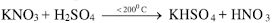(b) Concentrated hydrochloric acid cannot replace Conc. Sulphuric acid for the preparation of nitric acid because hydrochloric acid is volatile acid and hence nitric acid vapours will carry HCl vapours.

(c) Conc. Nitric acid prepared in the laboratory is yellow in colour due to the dissolution of
reddish-brown coloured nitrogen dioxide gas in acid. This gas is produced due to the thermal dissociation of a portion of nitric acid.
4HNO3  + 2H2O ⟶ 4NO2 + O2
The yellow colour of the acid is removed:
If dry air or CO2 is bubbled through the yellow acid, the acid turns colourless because it drives out NO2  from warm acid which is further oxidized to nitric acid.
By addition of excess of water, nitrogen dioxide gas dissolves in water and thus the yellow colour of the acid is removed.

(d) The temperature of the mixture of concentrated sulphuric acid and sodium nitrate should not exceed 200°C because sodium sulphate formed at higher temperature forms a hard crust which sticks to the walls of the retort and is difficult to remove. At higher temperature, nitric acid may also decompose.Question 4: Nitric acid cannot be concentrated beyond 68% by the distillation of a dilute solution of HNO3 State the reason.

Solution 4: Nitric acid forms a constant boiling mixture with water containing 68% acid. This mixture boils constantly at constant boiling point without any change in its composition. At this temperature, the gas and the water vapour escape together. Hence the composition of the solution remains unchanged. So nitric acid cannot be concentrated beyond 68% by distillation of dilute solution of HNO3 .

Question 5: What is passive iron? How is passivity removed?

Solution 5: Iron becomes inert when reacted with nitric acid due to the formation of an extremely thin layer of insoluble metallic oxide which stops the reaction. Passivity can be removed by rubbing the surface layer with the sand paper or by treating with strong reducing agent.

Question 6: Name the products formed when:
(a) carbon and conc. Nitric acid is heated
(b) dilute HNO3  is added to copper.

Solution 6: (a) When carbon and conc. Nitric acid is heated the products formed are Carbon dioxide, Nitrogen dioxide and water.
C + 4HNO3 → CO2 + 2H2O + 4NO2

(b) Copper when reacts with dilute HNO3 forms Copper nitrate, Nitric oxide and water.
3Cu + 8HNO3 → 3Cu(NO3)2 + 4H2O + 2NO

Question 7: Give two chemical equations for each of the following:
(a) Reactions of nitric acid with non-metals
(b) Nitric acid showing as acidic character
(c) Nitric acid acting as oxidizing agent

Solution 7: (a) Reaction of nitric acid with non-metals:
C + 4HNO3 → CO2 + 2H2O + 4NO2
S + 6 HNO3 → H2SO4 + 2H2O + 6 NO2

(b) Nitric acid showing acidic character:
K2O + 2HNO3 → 2KNO3  + H2O
ZnO + 2HNO3 → Zn(NO3)2 + H2O

(c) Nitric acid acting as oxidizing agent
P4 + 2OHNO3 → 4H3PO4 + 4H2O + 2ONO2
3Zn + 8HNO3 → 3Zn(NO3)2 + 4H2O + 2NO

Question 8: Write balanced equations and name the products formed when:
(a) Sodium hydrogen carbonate is added to nitric acid
(b) cupric oxide reacts with nitric acid
(c) zinc reacts with dilute nitric acid
(d) concentrated nitric acid is heated

Solution 8: (a) When Sodium hydrogen carbonate is added to nitric acid sodium nitrate, carbon dioxide and water is formed.
NaHCO3 + HNO3 → NaNO3 + H2O + CO2

(b) When Cupric oxide reacts with dilute nitric acid, it forms Copper nitrate.
CuO + 2HNO3 → Cu(NO3)2 + H2O

(c) Zinc reacts with nitric acid to form Zinc nitrate, nitric oxide and water.
3 Zn + 8HNO3 → 3Zn(NO3)2 + 4H2O + 2NO
(d) 4HNO3 → 2H2O + 4NO2 + O2

Question 9: Write an equation for the following conversions A, B, C and D.Solution 9:
A: Copper can be converted into copper nitrate.
3Cu + 8HNO3 → 3Cu(NO3)2 + 4H2O + 2NO
B: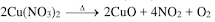C: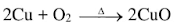D: By reduction
2CuO + C → 2Cu + CO2

Question 10: How will you prepare the following from nitric acid?
(a) Sodium nitrate
(b) copper nitrate
(d) Magnesium nitrate
(e) Ferric nitrate
(f) Aqua regia

Solution 10: (a) Sodium nitrate:
NaOH + HNO3  → NaNO3 + H2O
Sodium hydroxide reacts with nitric acid to form sodium nitrate.

(b) Copper nitrate:
CuO + 2HNO3 → Cu(NO3)2 + H2O
Copper oxide reacts with nitric acid to form copper nitrate.

Pb + 4HNO3 → Pb(NO3)2 + 2H2O + 2NO2

(d) Magnesium nitrate:
Mg + 2HNO3 → Mg(NO3)2 + H2
Magnesium with dil. nitric acid to form magnesium nitrate.

(e) Ferric nitrate:
Fe + 6HNO3 → Fe(NO3)3 + 3H2O + 3NO2
Iron reacts with conc. nitric acid to form ferric nitrate.

(f) Aqua regia:
HNO3 + 3HCl → NOCI + 2H2O + 2[Cl]
Nitric acid reacts with hydrochloric acid to form a mixture called aqua regia.

Question 11: Correct the following, if required:
(a) HNO3  is strong reducing agent.
(b) NaNO3  gives Na2  and O2 on heating.
(c) Constant boiling nitric acid contains 80% nitric acid by weight.
(d) Nitric acid remains colourless even when exposed to light.

Solution 11: (a) HNO3 is strong oxidizing agent.
(b) NaNO3   gives NaNO2 and oxygen on heating.
(c) Constant boiling nitric acid contains 68% nitric acid by weight.
(d) Nitric acid turns yellow solution when exposed to light.

Question 12: Name:
(a) a nitrate of metal which on heating does not give nitrogen dioxide
(b) a nitrate which on heating leaves no residue behind.
(c) a metal nitrate which on heating is changed into metal oxide
(d) a metal nitrate which on heating is changed into metal
(e) a solution which absorbs nitric oxide
(f) the oxide of nitrogen which turns brown on exposure to air. How is it prepared?

Solution 12: (a) Sodium nitrate(b) A nitrate which on heating leaves no residue behind- Ammonium nitrate.
(c) A metal nitrate which on heating is changed into metal oxide- Calcium nitrate
(d) A metal nitrate which on heating is changed into metal - Silver nitrate
(e) A solution which absorbs nitric oxide- Freshly prepared ferrous sulphate
(f) The oxide of nitrogen which turns brown on exposure to air. - nitric oxide
By catalytic oxidation of ammonia.Question 13: Give the chemical name and formula of the substance formed as a brown ring in the test for nitrate radical.

Solution 13: The chemical name of the brown ring is Nitroso ferrous sulphate.
Formula: FeSO4. NO

Question 14: Mention three important uses of nitric acid. Give the property of nitric acid involved in the use.

Solution 14: Three important uses of Nitric acid and the property of nitric acid involved is:
 Sl. No. Use Property 1. To etch designs on copper and brassware. Nitric acid act as solvent for large number of metals. 2. To purify gold. Impurities like Cu, Ag, Zn, etc. dissolve in nitric acid. 3. Preparation of aqua regia. Dissolves noble metals.

Question 15: Choose the correct answer:
(a) The nitrate salt which does not give a mixture of NO2 and O2 on heating is:
(i) AgNO3 (ii) KNO3 (iii) Cu(NO3)2 (iv) Zn(NO3)2

(b) The chemical used in the brown ring test is:
(i) CuSO4 (ii) FeSO(iii) Fe2(SO4)3 (iv) NO3O5

Solution 15: (a) (ii) KNO3
(b) (ii) FeSO4
(c) NO2

Question 16: (a) Explain with the help of a balanced equation, the brown ring test for nitric acid.
(b) why is freshly prepared ferrous sulphate solution used for testing the nitrate radical in the brown ring test?

Solution 16: (a) Brown ring test
Procedure:
(i) Add freshly prepared saturated solution of iron (II) sulphate to the aq. solution of nitric acid.
(ii) Now add conc. Sulphuric acid carefully from the sides of the test tube, so that it should not fall drop wise in the test tube.
(iii) Cool the test tube in water.
(iv) A brown ring appears at the junction of the two liquids.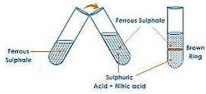6FeSO4 + 2HNO3 + 3H2SO4 → 3Fe2 (SO4)3 + 2NO + 4H2O

(b) A freshly prepared ferrous sulphate solution is used because on exposure to the atmosphere, it is oxidized to ferric sulphate which will not give the brown ring.

Question 17: From the following list of substances, choose one substance in each case which matches the description given below:
Ammonium nitrate, calcium hydrogen carbonate, copper carbonate, lead nitrate, potassium nitrate, sodium carbonate, sodium hydrogen carbonate, zinc carbonate.
(a) A nitrate which gives off only oxygen when heated
(b) A nitrate which on heating decomposes into dinitrogen oxide (nitrous oxide) and steam.
(c) A nitrate which gives off oxygen and nitrogen dioxide when heated.

Solution 17: (a) Potassium nitrate
(b) Ammonium nitrate

Question 18: Fill in the blanks:
(a) Aqua regia is a mixture of 3 parts ---------- and one part ----------
(b) The catalytic oxidation of ammonia to nitric oxide is----------- (exothermic/endothermic) process.
(c) Magnesium gives ----------(O2, H2, NO) with very dilute nitric acid.
(d) ----------- (iron/copper) become passive in concentrated nitric acid

Solution 18: (a) Aqua regia is a mixture of 3 parts Hydrochloric acid and one part Nitric acid.
(b) The catalytic oxidation of ammonia to nitric oxide is exothermic.
(c) Magnesium gives H2 with very dilute nitric acid.
(d) Iron becomes passive in concentrated nitric acid

Question 19: The action of heat on the blue crystalline solid A, gives a reddish-brown gas B, a gas which relights a glowing splint and leaves a black residue. When gas C, which has a rotten egg smell, is passed through a solution of A, a black ppt. is formed.
(a) Identify A, B and C
(b) Write an equation for action of heat on A.
(c) Write the equation between the solution of A and gas C.

Solution 19: (a) A= copper nitrate, B = nitrogen dioxide, C = hydrogen sulphide
(b) 2Cu(NO3)2 ⟶ 2CuO + 4NO2 + O2
(c) Cu(NO3)2 + H2S ⟶ CuS + 2HNO3

Question 20: X, Y and Z are three crystalline solids which are soluble in water and have a common anion. To help you to identify X, Y and Z you are provided with the following experimental observations. Copy and complete the corresponding inferences in (a) to (f).
(a) A reddish-brown gas is obtained when X, Y and Z are separately warmed with concentrated sulphuric acid and copper turning added to the mixture.

INFERENCE 1 : The common anion is the.....................ion.
(b) When X is heated, it melts and gives off only one gas which re-lights a glowing splint.

INFERENCE 2: The cation in X is either
(c) The action of heat on Y produces a reddish-brown gas and a yellow residue which fuses with the glass of the test tube.

INFERENCE 3: The metal ion present in Y is the ....................... ion
(d) When Z is heated, it leaves no residue. Warming Z with sodium hydroxide solution liberates a gas which turns moist red litmus paper blue.

INFERENCE 4: Z contains the .................... Cation.
(e) Write the equations for the following reactions:
(1) X and concentrated sulphuric acid (below 200° C). (One equation only for either of the cations given in INFERENCE 2).
(2) Action of heat on Y.
(3) Concentrated nitric acid is added to copper turnings kept in a beaker.

Solution 20: (a) Nitrate.
(b) Sodium or potassium
(d) Ammonia

(e)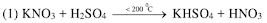(2)(3) Cu + 4HNO3 → Cu(NO3)2 + H2O + 2N2O

Question 21: (a) Dilute acid is generally considered a typical acid except for its reaction with metals. In what way is dilute nitric acid different from other acids when it reacts with metals?
(b) Write the equation for the reaction of dilute nitric acid with copper.

Solution 21: (a) Dilute acid is generally considered a typical acid except for its reaction with metals since it does not liberate hydrogen. It is a powerful oxidizing agent and the nascent oxygen formed oxidizes the hydrogen to water.

(b) 3Cu + 8HNO3 → 3Cu(NO3)2 + 4H2O + 2NO

Question 22: Explain why:
(a) Only all-glass apparatus should be used for the preparation of nitric acid by heating concentrated sulphuric acid and potassium nitrate.
(b) Nitric acid is kept in a reagent bottle for a long time.

Solution 22: (a) All glass apparatus are used because nitric acid vapours are highly corrosive in nature and corrode cork and rubber etc.
(b) Nitric acid is kept in reagent bottle because nitric acid is a highly fuming liquid; it spreads in air and is highly corrosive.

Question 23: Write a chemical equation to illustrate the acidic nature of nitric acid.

Solution 23: The chemical equation:
HNO3 ⇌ H+ + NO3-

Question 24: Name the products formed when ammonium nitrate is heated.

Solution 24: When ammonium nitrate is heated the products formed are nitrous oxide and steam.

Question 25: The figure given below illustrates the apparatus used in the laboratory preparation of nitric acid.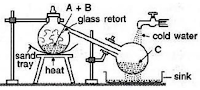(a) Name A (a liquid), B (a solid) and C (a liquid). ( Do not give the formulae)
(b) Write an equation to show how nitric acid undergoes decomposition
(c) Write the equation for the reaction in which copper is oxidized by concentrated nitric acid

Solution 25: (a) A = Conc. Sulphuric acid
B = potassium nitrate
C = nitric acid

(b) Nitric acid undergoes decomposition as follows: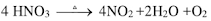(c) Copper is oxidized by concentrated nitric acid:
Cu + 4HNO3 → Cu(NO3)+ 2H2O + 2NO2

Question 26: (a) A dilute acid B does not normally give hydrogen when reacted with metals but does give a gas when reacts with copper. Identify B. Write equation with copper.
(b) Complete the table:(c) What is the property of nitric acid which allows it to reacts with copper?

Solution 26: (a) B is nitric acid
Its reaction with copper:(b)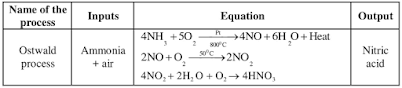(c) Nitric acid is a very good oxidizing agent its oxidizing property is responsible for its reaction with copper.